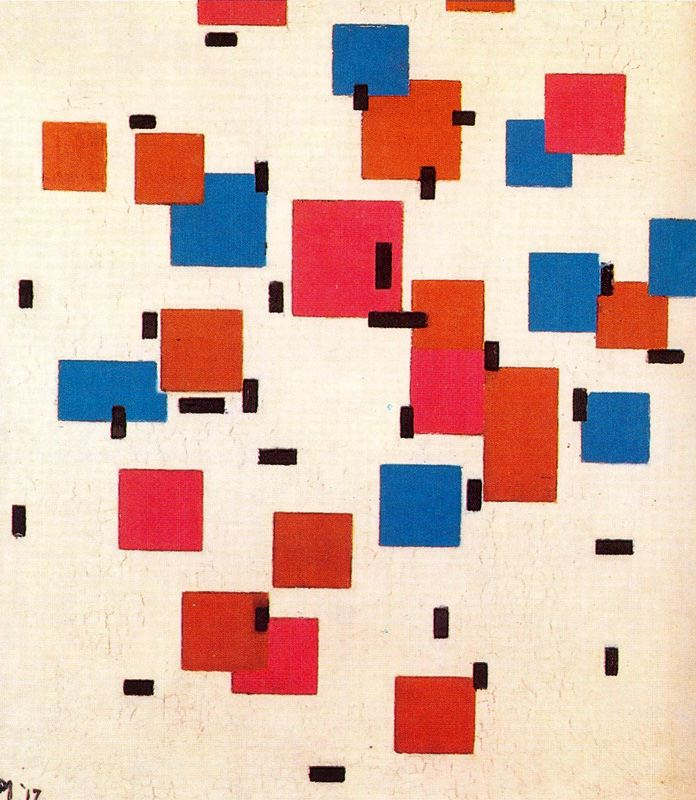# [Java] Introduction to Programming for College Students: How to Draw a Basic Rectangle

• With Qiita, it is difficult to print only the text part. Therefore, the same contents are also posted on the following pages. If you want to print out or convert to PDF, please use this page (same content):

http://gurakura.sakura.ne.jp/hellomondrian/rect1/

# Introduction: draw a rectangleThe above image is Piet Mondrian’s composition Composition in Color A (1917), [https://www.wikiart.org/en/piet-mondrian/composition-in-color-a-1917](https://(www.wikiart.org/en/piet-mondrian/composition-in-color-a-1917) Can be seen at.

I explained how to draw straight lines in the previous chapter. If you just draw the outline of a rectangle, you can call the line function four times to draw.

In this chapter, we will explain not how to draw, but how to draw a rectangle that fills the inside, that is, a rectangle as a region.

# How to draw a basic rectangle

Use the rect function to draw a rectangle in which horizontal and vertical lines are parallel to the window. The argument of the rect function is rect(x,y,w,h), and

x = the x coordinate of the upper left corner of the rectangle y = y coordinate of the upper left corner of the rectangle w = width of rectangle h = height of rectangle

Will be.

The drawing of the rectangle by rect is affected by the stroke width setting and stroke stroke color.

``````background(250,250,250);
size(500,500);

strokeWeight(10); // line width: 10 pixels.
stroke(0,64,255); // line color: light blue.
rect(100,150,200,100);
``````The drawing color inside the rectangle can be specified with the fill function. This function has various uses as well as the stroke function, but the method of specifying RGB value fill(r,g,b) is introduced here.

In the program list shown below, the outline is blue, as in the previous program, and the inside is specified to be filled with red.

``````background(250,250,250);
size(500,500);

strokeWeight(10); // line width: 10 pixels.
stroke(0,64,255); // line color: light blue.

fill(255,0,0); // <--- fill color: red.

rect(100,150,200,100);
``````As you can see, in drawing a rectangle, you have to manage the outline color and the two colors that fill the inside.

It may seem awkward, but programming is a series of such tasks. Well, it’s sober and detailed, and it’s a painful task. So, please be kind to programmers (no, really…).

As the end of this section, I would like to consider a method that does not draw outlines. What do you think should be done? I think there are various ideas.

Should I instruct Processing not to draw the contour line in the first place? Some people thought that if the width of the contour line is set to 0, it will disappear. Etc., etc. Both of these opinions are correct.

Thus, in programming, there are multiple ways to achieve the same effect.

Of course, the speed of execution may differ in some cases, and there are few things that are exactly the same.

However, in real-world programming, there are often different ways to reach the same goal.

There is a noStroke function as a function that turns off the contour line. Since this is a function that does not require any arguments, use it as stroke(); in a real program. To enable the disabled contour, specify the contour color with the stoke function.

Even if you turn off the outline with the noStroke function, the information about the width of the outline remains, so the square outline drawn by the second rect function remains 10 pixels.

``````background(250,250,250);
size(500,500);

strokeWeight(10); // line width: 10 pixels.
stroke(0,64,255); // set color, but disabled by noStroke
fill(255,0,0);
noStroke();
rect(100,150,200,100);

stroke(0,64,255); // stroke ON (line color: light blue.)
rect(100,400,50,80);
``````Tags:

Updated: